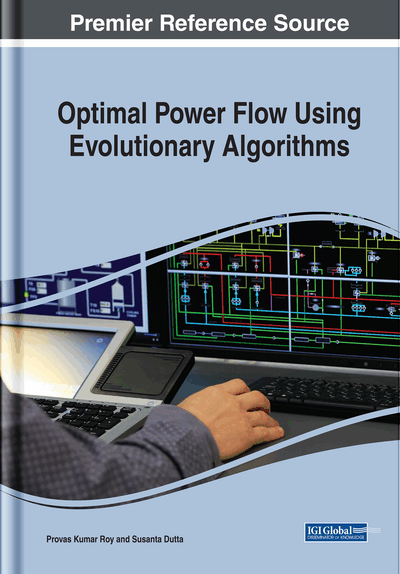# Description of Various Evolutionary Optimization Techniques

DOI: 10.4018/978-1-5225-6971-8.ch001
Available
\$37.50
No Current Special Offers

## Abstract

Despite the success of various classical optimization techniques, there remains a large class of problems where either these methods are unable to find the satisfactory results or the computational times are sufficiently large. Several heuristic methods have emerged in the recent years as complementary tools to various mathematical approaches. These methods include genetic algorithm (GA), evolutionary programming (EP), particle swarm optimization (PSO), differential evolution (DE), and so on. Researchers are constantly trying to learn from the behavioral pattern of organisms and implementing those ideas and philosophies in solving optimizing problems. In this chapter, a few efficient optimization algorithms, namely grey wolf optimization (GWO), teaching-learning-based optimization (TLBO), biogeography-based optimization (BBO), krill herd algorithm (KHA), chemical reaction optimization (CRO) algorithms, and hybrid CRO (HCRO) are discussed, and in the subsequent chapters, the performance of the aforesaid algorithms are investigated by applying them in a few areas of power systems.
Chapter Preview
Top

## Introduction

We often encounter optimization problems in scientific and technological research and development. The word “optimum” is latin, and means “the ultimate ideal” similarly, “optimus” means “the best.” Therefore, to optimize refers to try to bring whatever optimize we are dealing with towards its ultimate state. Let us take a closer look at what that means in terms of an example, and at the same time bring the definition of the term optimization forward, as the scientific field understands and uses it. Optimization is the process of making something better. In other words, optimization is the process of adjusting the inputs to or characteristics of a device, mathematical process, or experiment to find the minimum or maximum output or result.

Optimized operation of the power system is a challenging problem due to its complex and non-linear nature. There are also many uncertainties in power system, which add its complexity further. Recently, with the introduction of deregulation in power industry (Roy, 2018) many new complexities are added to the existing problems. The application of optimization techniques to various power system problems has been an area of research for many years.

The different techniques are applied to power system optimization problems over the years. These algorithms are generally divided into two categories: (i) classical mathematical optimization algorithms and (ii) intelligent optimization algorithms. The basic idea of the classical algorithm is starting from an initial point, continuously improving the current solution through a certain orbit, and ultimately converging to the optimal solution. These algorithms include linear programming (LP) (Aoki et al., 1988; Deeb, & Shahidehpour, 1988; Momoh et al., 1999; Urdaneta et al., 1999), Lagrangian decomposition method (Granada et al., 2012), quadratic programming (QP) (Grudinin, 1998; Lo & Zhu, 1991), non-linear programming (NLP) (Habiabollahzadeh et al., 1989; Lai & Ma, 1997), interior point method (IPM) (Capitanescu & Wehenkel, 2013; Granville, 1995; Yan. & Quintana, 1999; Zhu & Xiong, 2003) Newton method (Santos & Costa, 1995; Sun et al., 1984), mixed integer linear programming (MILP) (Kim et al., 1998), gradient search (Burchett, 2013; Yu et al., 1986), neural network (NN) (Dhillon, 1991; Park et al., 1993), Lagrange Hopfield network (Vo & Ongsakul, 2012), etc. Some of these techniques, however, have a good convergence, but most of them suffer from reaching the global optimal solution because the most of the power system optimization problems are a multimodal and non-linear problem and severely depends on the initial guess.

## Complete Chapter List

Search this Book:
Reset